# 浅谈Kotlin中的Sequences源码解析(十)• 1、为什么需要序列(Sequences)?
• 2、什么是序列(Sequences)?
• 3、怎么创建序列(Sequences)?
• 4、序列(Sequences)操作和集合操作性能对比
• 5、序列(Sequences)性能优化的原理
• 6、序列(Sequences)原理源码完全解析

### 1、为什么需要序列(Sequences)?

``````//不使用Sequences序列，使用普通的集合操作
fun computeRunTime(action: (() -> Unit)?) {
val startTime = System.currentTimeMillis()
action?.invoke()
println("the code run time is \${System.currentTimeMillis() - startTime}")
}

fun main(args: Array<String>) = computeRunTime {
(0..10000000)
.map { it + 1 }
.filter { it % 2 == 0 }
.count { it < 10 }
.run {
println("by using list way, result is : \$this")
}
}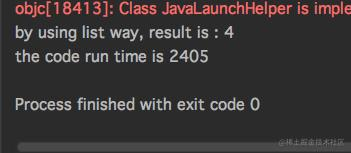``````//转化成Sequences序列，使用序列操作
fun computeRunTime(action: (() -> Unit)?) {
val startTime = System.currentTimeMillis()
action?.invoke()
println("the code run time is \${System.currentTimeMillis() - startTime}")
}

fun main(args: Array<String>) = computeRunTime {
(0..10000000)
.asSequence()
.map { it + 1 }
.filter { it % 2 == 0 }
.count { it < 10 }
.run {
println("by using sequences way, result is : \$this")
}
}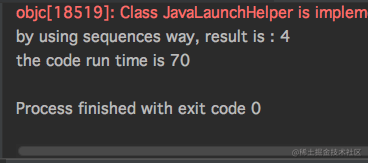### 2、什么是序列(Sequences)?

• 1、中间操作

``````fun main(args: Array<String>) {
(0..6)
.asSequence()
.map {//map返回是Sequence<T>，故它属于中间操作
println("map: \$it")
return@map it + 1
}
.filter {//filter返回是Sequence<T>，故它属于中间操作
println("filter: \$it")
return@filter it % 2 == 0
}
}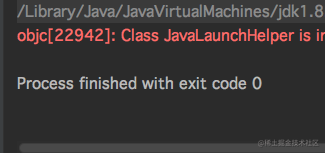• 2、末端操作

``````fun main(args: Array<String>) {
(0..6)
.asSequence()
.map {//map返回是Sequence<T>，故它属于中间操作
println("map: \$it")
return@map it + 1
}
.filter {//filter返回是Sequence<T>，故它属于中间操作
println("filter: \$it")
return@filter it % 2 == 0
}
.count {//count返回是Int，返回的是一个结果，故它属于末端操作
it < 6
}
.run {
println("result is \$this");
}
}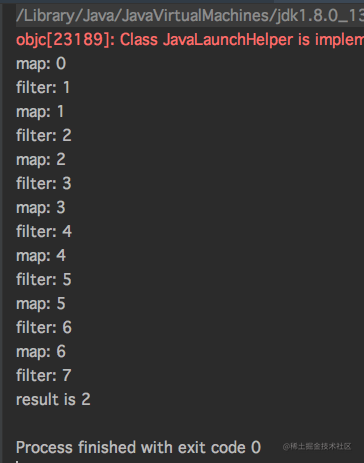### 3、怎么创建序列(Sequences)?

• 1、使用Iterable的扩展函数asSequence来创建。
``````//定义声明
public fun <T> Iterable<T>.asSequence(): Sequence<T> {
return Sequence { this.iterator() }
}
//调用实现
list.asSequence()

• 2、使用generateSequence函数生成一个序列。
``````//定义声明
public fun <T : Any> generateSequence(seed: T?, nextFunction: (T) -> T?): Sequence<T> =
if (seed == null)
EmptySequence
else
GeneratorSequence({ seed }, nextFunction)

//调用实现，seed是序列的起始值，nextFunction迭代函数操作
val naturalNumbers = generateSequence(0) { it + 1 } //使用迭代器生成一个自然数序列

• 3、使用序列(Sequence<T>)的扩展函数constrainOnce生成一次性使用的序列。
``````//定义声明
public fun <T> Sequence<T>.constrainOnce(): Sequence<T> {
// as? does not work in js
//return this as? ConstrainedOnceSequence<T> ?: ConstrainedOnceSequence(this)
return if (this is ConstrainedOnceSequence<T>) this else ConstrainedOnceSequence(this)
}
//调用实现
val naturalNumbers = generateSequence(0) { it + 1 }
val naturalNumbersOnce = naturalNumbers.constrainOnce()

### 4、序列(Sequences)操作和集合操作性能对比

• 1、同样数据操作在数据量级比较大情况下。

``````fun computeRunTime(action: (() -> Unit)?) {
val startTime = System.currentTimeMillis()
action?.invoke()
println("the code run time is \${System.currentTimeMillis() - startTime} ms")
}

fun main(args: Array<String>) = computeRunTime {
(0..10000000)//10000000数据量级
.asSequence()
.map { it + 1 }
.filter { it % 2 == 0 }
.count { it < 100 }
.run {
println("by using sequence result is \$this")
}
}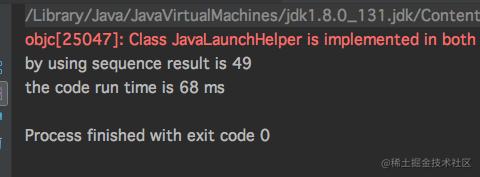``````fun computeRunTime(action: (() -> Unit)?) {
val startTime = System.currentTimeMillis()
action?.invoke()
println("the code run time is \${System.currentTimeMillis() - startTime} ms")
}

fun main(args: Array<String>) = computeRunTime {
(0..10000000)//10000000数据量级
.map { it + 1 }
.filter { it % 2 == 0 }
.count { it < 100 }
.run {
println("by using sequence result is \$this")
}
}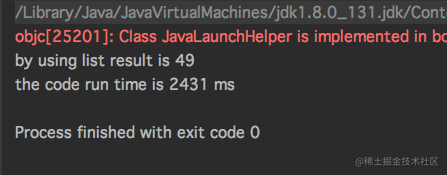• 2、同样数据操作在数据量级比较小情况下。

``````fun computeRunTime(action: (() -> Unit)?) {
val startTime = System.currentTimeMillis()
action?.invoke()
println("the code run time is \${System.currentTimeMillis() - startTime} ms")
}

fun main(args: Array<String>) = computeRunTime {
(0..1000)//1000数据量级
.asSequence()
.map { it + 1 }
.filter { it % 2 == 0 }
.count { it < 100 }
.run {
println("by using sequence result is \$this")
}
}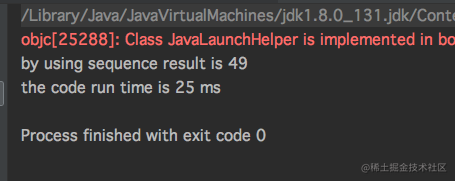``````fun computeRunTime(action: (() -> Unit)?) {
val startTime = System.currentTimeMillis()
action?.invoke()
println("the code run time is \${System.currentTimeMillis() - startTime} ms")
}

fun main(args: Array<String>) = computeRunTime {
(0..1000)//1000数据量级
.map { it + 1 }
.filter { it % 2 == 0 }
.count { it < 100 }
.run {
println("by using list result is \$this")
}
}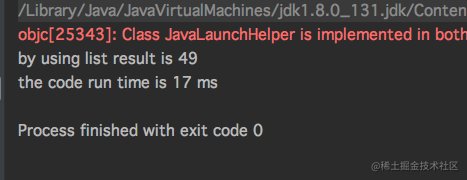### 5、序列(Sequences)性能优化的原理

``````fun main(args: Array<String>){
(0..10)
.asSequence()
.map { it + 1 }
.filter { it % 2 == 0 }
.count { it < 6 }
.run {
println("by using sequence result is \$this")
}
}

• 1、基本原理描述

• 2、原理图解
``````//使用序列
fun main(args: Array<String>){
(0..100)
.asSequence()
.map { it + 1 }
.filter { it % 2 == 0 }
.find { it > 3 }
}
//使用普通集合
fun main(args: Array<String>){
(0..100)
.map { it + 1 }
.filter { it % 2 == 0 }
.find { it > 3 }
}

### 6、序列(Sequences)原理源码完全解析

``````//使用序列
fun main(args: Array<String>){
(0..100)
.asSequence()
.map { it + 1 }
.filter { it % 2 == 0 }
.find { it > 3 }
}
//使用普通集合
fun main(args: Array<String>){
(0..100)
.map { it + 1 }
.filter { it % 2 == 0 }
.find { it > 3 }
}

#### 使用集合普通操作反编译源码

`````` public static final void main(@NotNull String[] args) {
Intrinsics.checkParameterIsNotNull(args, "args");
byte var1 = 0;
Iterable \$receiver\$iv = (Iterable)(new IntRange(var1, 100));
//创建新的集合存储map后中间结果
Collection destination\$iv\$iv = (Collection)(new ArrayList(CollectionsKt.collectionSizeOrDefault(\$receiver\$iv, 10)));

int it;
//对应map操作符生成一个while循环
while(var4.hasNext()) {
it = ((IntIterator)var4).nextInt();
Integer var11 = it + 1;
//将map变换的元素加入到新集合中
}

//创建新的集合存储filter后中间结果
destination\$iv\$iv = (Collection)(new ArrayList());
//对应filter操作符生成一个while循环
while(var4.hasNext()) {
Object element\$iv\$iv = var4.next();
int it = ((Number)element\$iv\$iv).intValue();
if (it % 2 == 0) {
//将filter过滤的元素加入到新集合中
}
}

//对应find操作符生成一个while循环,最后末端操作只需要遍历filter后新集合中的迭代器，取出符合条件数据即可。
while(var13.hasNext()) {
Object var14 = var13.next();
it = ((Number)var14).intValue();
if (it > 3) {
break;
}
}
}

#### 使用序列(Sequences)惰性操作反编译源码

• 1、整个序列操作源码
`````` public static final void main(@NotNull String[] args) {
Intrinsics.checkParameterIsNotNull(args, "args");
byte var1 = 0;
//利用Sequence扩展函数实现了fitler和map中间操作，最后返回一个Sequence对象。
Sequence var7 = SequencesKt.filter(SequencesKt.map(CollectionsKt.asSequence((Iterable)(new IntRange(var1, 100))), (Function1)null.INSTANCE), (Function1)null.INSTANCE);
//取出经过中间操作产生的序列中的迭代器，可以发现进行map、filter中间操作共享了同一个迭代器中数据，每次操作都会产生新的迭代器对象，但是数据是和原来传入迭代器中数据共享，最后进行末端操作的时候只需要遍历这个迭代器中符合条件元素即可。
Iterator var3 = var7.iterator();
//对应find操作符生成一个while循环,最后末端操作只需要遍历filter后新集合中的迭代器，取出符合条件数据即可。
while(var3.hasNext()) {
Object var4 = var3.next();
int it = ((Number)var4).intValue();
if (it > 3) {
break;
}
}

}

• 2、抽出其中这段关键code，继续深入:
``````SequencesKt.filter(SequencesKt.map(CollectionsKt.asSequence((Iterable)(new IntRange(var1, 100))), (Function1)null.INSTANCE), (Function1)null.INSTANCE);

• 3、把这段代码转化分解成三个部分:
``````//第一部分
val collectionSequence = CollectionsKt.asSequence((Iterable)(new IntRange(var1, 100)))
//第二部分
val mapSequence = SequencesKt.map(collectionSequence, (Function1)null.INSTANCE)
//第三部分
val filterSequence = SequencesKt.filter(mapSequence, (Function1)null.INSTANCE)

• 4、解释第一部分代码:

``````public fun <T> Iterable<T>.asSequence(): Sequence<T> {
return Sequence { this.iterator() }//传入外部Iterable<T>中的迭代器对象
}

``````@kotlin.internal.InlineOnly
public inline fun <T> Sequence(crossinline iterator: () -> Iterator<T>): Sequence<T> = object : Sequence<T> {
override fun iterator(): Iterator<T> = iterator()
}

• 5、解释第二部分的代码:

``````val mapSequence = SequencesKt.map(collectionSequence, (Function1)null.INSTANCE)

``````public fun <T, R> Sequence<T>.map(transform: (T) -> R): Sequence<R> {
return TransformingSequence(this, transform)
}

• 6、解释第三部分的代码:

``````val filterSequence = SequencesKt.filter(mapSequence, (Function1)null.INSTANCE)

``````public fun <T> Sequence<T>.filter(predicate: (T) -> Boolean): Sequence<T> {
return FilteringSequence(this, true, predicate)
}

• 7、Sequences源码整体结构介绍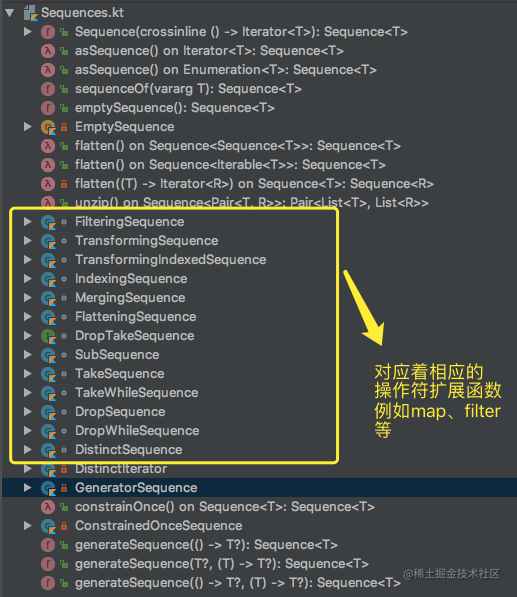``````public interface Sequence<out T> {
/**
* Returns an [Iterator] that returns the values from the sequence.
*
* Throws an exception if the sequence is constrained to be iterated once and `iterator` is invoked the second time.
*/
public operator fun iterator(): Iterator<T>
}

Sequence核心类UML类图

• 8、接着上面TransformingSequence、FilteringSequence继续解析.

``````//实现了Sequence<R>接口，重写了iterator()方法，重写迭代器的实现
internal class TransformingSequence<T, R>
constructor(private val sequence: Sequence<T>, private val transformer: (T) -> R) : Sequence<R> {
override fun iterator(): Iterator<R> = object : Iterator<R> {//根据传入的迭代器对象中的数据，加以操作变换后，构造出一个新的迭代器对象。
val iterator = sequence.iterator()//取得传入Sequence中的迭代器对象
override fun next(): R {
return transformer(iterator.next())//将原来的迭代器中数据元素做了transformer转化传入，共享同一个迭代器中的数据。
}

override fun hasNext(): Boolean {
return iterator.hasNext()
}
}

internal fun <E> flatten(iterator: (R) -> Iterator<E>): Sequence<E> {
return FlatteningSequence<T, R, E>(sequence, transformer, iterator)
}
}

• 9、源码分析总结Android